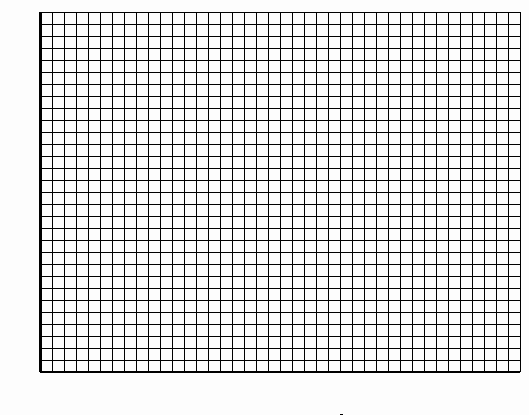PRINT THIS ANSWER SHEET TO FILL OUT AND TURN IN.

NAME_________________________________ CLASS____________________ DATE____________

## Lift vs. Airspeed Worksheet

Step 2.

Using the chart below, record the Airspeed and the Lift. By changing the Airspeed, make at least 9 additional readings. Record these in the chart.

 Airspeed (km/hr) Lift (newtons) 0

Step 3.

Enter your data into the Lists in your calculator. Set up Stat Plot so that the Airspeed is your independent variable and the Lift is the dependent variable. Graph the data on your calculator and make a sketch in the graph below.Step 4.

In FoilSim, push the Plots output button and select Lift vs. Speed. How does this graph compare to yours?

Step 5.

Which kind of function does this graph represent?

Step 6.

Using the regression equations (http://www.ti.com/calc/docs/act/pdf/marcus09.pdf) from your calculator, determine the equation of best fit.

a = ___________________

b= ___________________

c= ___________________

R2 = ___________________

equation: _______________________________

Step 7.

What does this equation tell you about the relationship between the change in Lift and the change in Airspeed?

Step 8.

Using the graph and/or the equation, what would you predict the lift to be given an airspeed of 300 km/hr.?